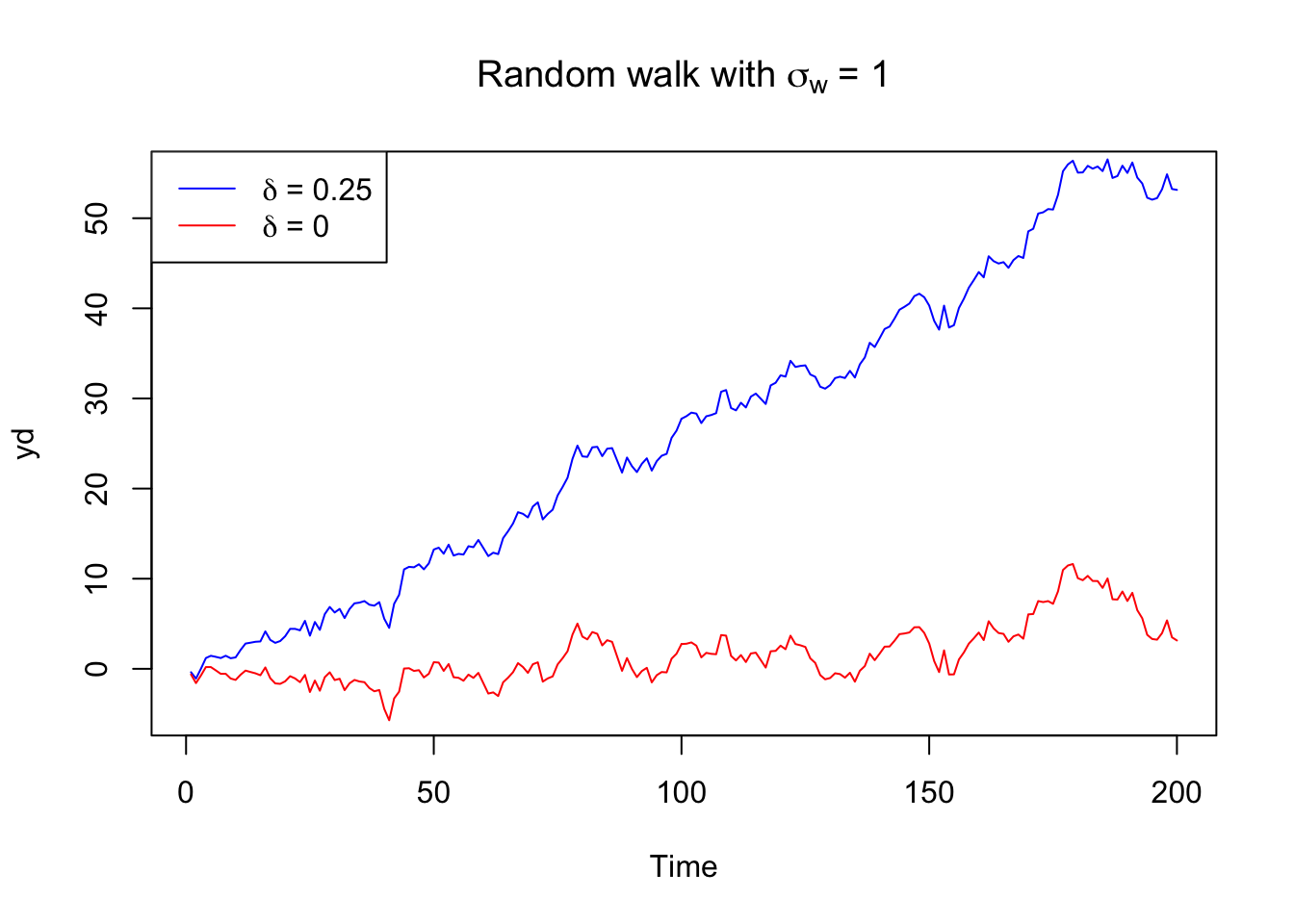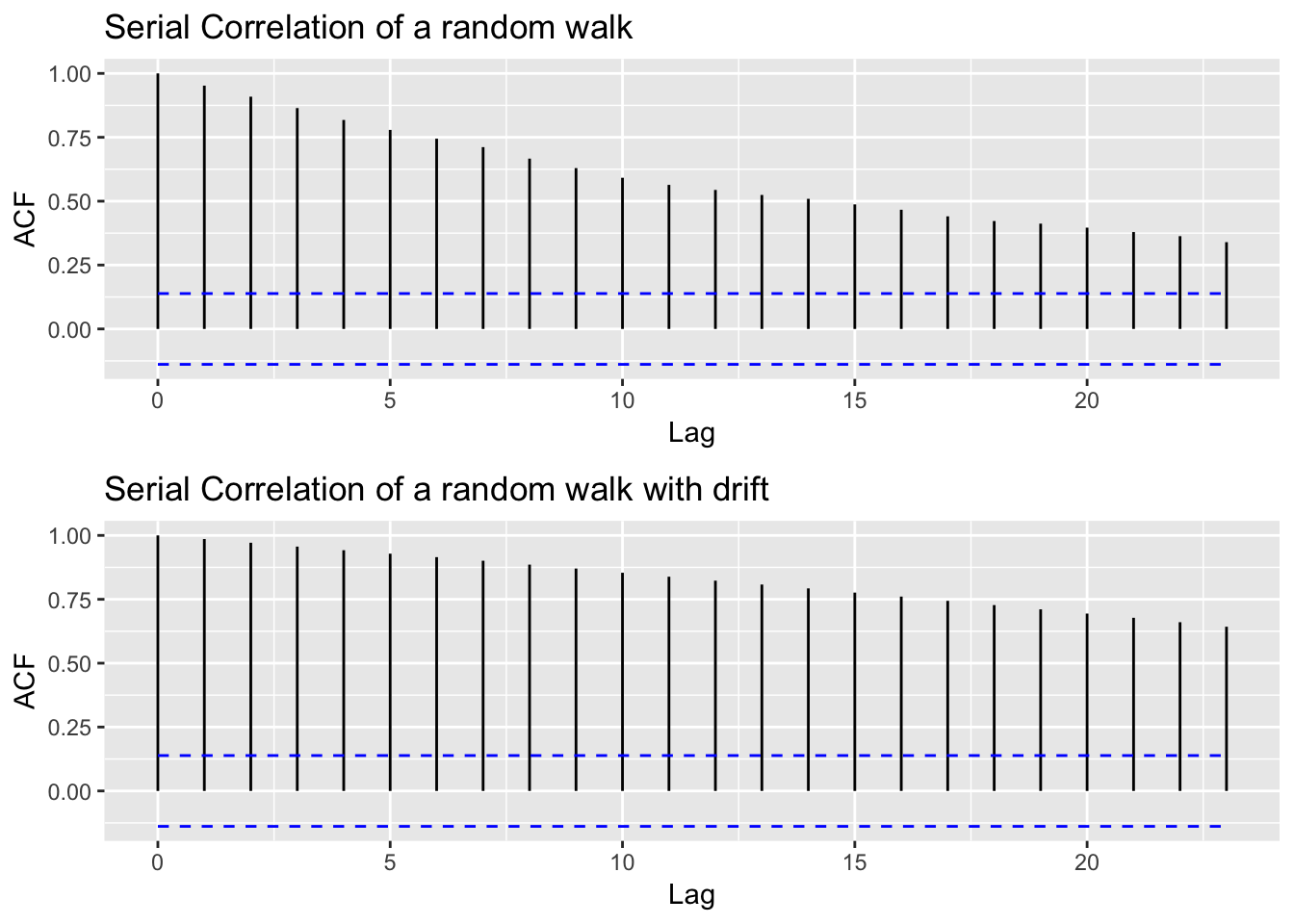A model for analyzing a series, which is the sum of a deterministic trend series and a stationary noise series is the random walk with drift model given by

$y_t = \delta+y_{t-1}+w_t$

for $$t = 1, 2,...$$ with initial condition $$y_0 = 0$$, and where $$w_t$$ is white noise. The constant $$\delta$$ is called the drift, and when $$\delta = 0$$ it is called simply a random walk.

The equation from above may be rewritten as

$y_t = \delta t + \sum_{j=1}^t w_j\text{,}$

for $$t = 1,2,...$$.

When $$\delta = 0$$ the value of the time series at time $$t$$ is the value of the series at time $$t-1$$ plus a completely random movement determined by $$w_t$$.

$\Delta y_t = y_t-y_{t-1} = w_t$

The $$w_{t}$$ values can be interpreted as independent “shocks” which perturb the current state $$y_t$$ by an amount $$w_{t}$$ to produce a new state $$y_{t+1}$$.

The graph below shows a random walk and a random walk with drift ($$\delta = 0.25$$) generated with R.

set.seed(250)
## random walk
w <- rnorm(n = 200, mean = 0, sd = 1)
y <- cumsum(w)
## random walk with drift
wd <- w +.25
yd <- cumsum(wd)
## plotting
plot.ts(yd, ylim = c(-5,55),
main = expression(paste("Random walk with " *sigma[w]* " = 1")),
col = 'blue')
lines(y, col = 'red')
legend('topleft',
legend = c(expression(paste(delta*' = 0.25')),
expression(paste(delta*' = 0'))),
col = c('blue', 'red'),
lty = 1)The autocorrelation function of this model type typically shows a very slow decay.

library(ggfortify)
library(gridExtra)

p1 <- autoplot(acf(y, plot = F)) +
ggtitle('Serial Correlation of a random walk')

p2 <- autoplot(acf(yd, plot = F)) +
ggtitle('Serial Correlation of a random walk with drift')
grid.arrange(p1, p2, ncol = 1)